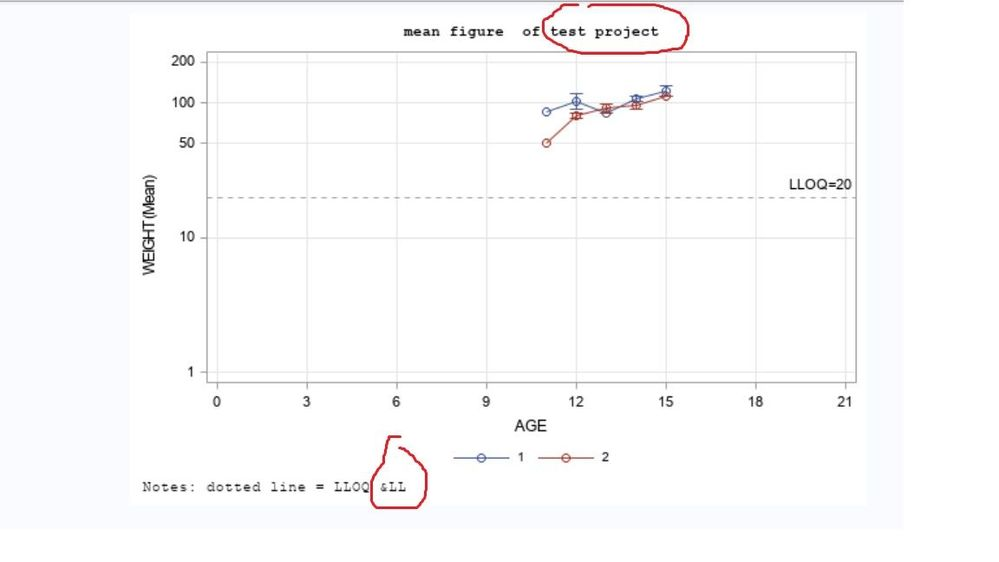## sgplot, how to add macro variable in footnote?

in sgplot, how to add macro variable in footnote?

I add macro variables in title and footnote, the title shows but footnote doesn't. I don't know why. anyone can help?

thanks a lot. below is my code.``````proc sort data=sashelp.class out=class;
by sex;
run;

data c1;
set class;
if sex='M' then do; lloq=5.1; unit='mg/kg';sexn=1; end;
if sex='F' then do; lloq=3; unit='mg/kg'; sexn=2; end;

run;

%MACRO ODS;
ods rtf file="c:\mean_plot.rtf"     STYLE=Styles.Stat     ;
%MEND;
%ODS;

%MACRO c1(ALLTEST,LL);

TITLE H=1 font= 'Courier New' c=black "mean figure  of &ALLTEST";
footnote H=1 j=l font= 'Courier New' c=black 'Notes: dotted line = LLOQ &LL';

proc sgplot data=c1;

where 11<=age<=15;
vline age/response=WEIGHT group=sexn grouporder=ascending stat=mean limitstat=stderr name="a"  markers;
refline &LL/axis=y label="LLOQ=&ll" LABELLOC= INSIDE lineattrs= (pattern=2);
xaxis type= linear GRID values=( 0 to 21 by 3);
yaxis type=log  GRID values=( 1 10 50 100 200) display=all    logstyle=logexpand minor ;
keylegend 'a'/noborder;

run;

%MEND;
%c1(ALLTEST=test project,LL=20);
ods rtf CLOSE;

``````
1 ACCEPTED SOLUTION

Accepted Solutions

## Re: sgplot, how to add macro variable in footnote?

Double quotes. Macro variables on resolve in double quotes,  not single quotes.

Use single quotes if you have text that has an & or % sign in it . Otherwise, in double quotes, SAS will try to interpret that as a macro or macro variable.

@magicdj wrote:

in sgplot, how to add macro variable in footnote?

I add macro variables in title and footnote, the title shows but footnote doesn't. I don't know why. anyone can help?

thanks a lot. below is my code.``````proc sort data=sashelp.class out=class;
by sex;
run;

data c1;
set class;
if sex='M' then do; lloq=5.1; unit='mg/kg';sexn=1; end;
if sex='F' then do; lloq=3; unit='mg/kg'; sexn=2; end;

run;

%MACRO ODS;
ods rtf file="c:\mean_plot.rtf"     STYLE=Styles.Stat     ;
%MEND;
%ODS;

%MACRO c1(ALLTEST,LL);

TITLE H=1 font= 'Courier New' c=black "mean figure  of &ALLTEST";
footnote H=1 j=l font= 'Courier New' c=black 'Notes: dotted line = LLOQ &LL';

proc sgplot data=c1;

where 11<=age<=15;
vline age/response=WEIGHT group=sexn grouporder=ascending stat=mean limitstat=stderr name="a"  markers;
refline &LL/axis=y label="LLOQ=&ll" LABELLOC= INSIDE lineattrs= (pattern=2);
xaxis type= linear GRID values=( 0 to 21 by 3);
yaxis type=log  GRID values=( 1 10 50 100 200) display=all    logstyle=logexpand minor ;
keylegend 'a'/noborder;

run;

%MEND;
%c1(ALLTEST=test project,LL=20);
ods rtf CLOSE;

``````

## Re: sgplot, how to add macro variable in footnote?

Double quotes. Macro variables on resolve in double quotes,  not single quotes.

Use single quotes if you have text that has an & or % sign in it . Otherwise, in double quotes, SAS will try to interpret that as a macro or macro variable.

@magicdj wrote:

in sgplot, how to add macro variable in footnote?

I add macro variables in title and footnote, the title shows but footnote doesn't. I don't know why. anyone can help?

thanks a lot. below is my code.``````proc sort data=sashelp.class out=class;
by sex;
run;

data c1;
set class;
if sex='M' then do; lloq=5.1; unit='mg/kg';sexn=1; end;
if sex='F' then do; lloq=3; unit='mg/kg'; sexn=2; end;

run;

%MACRO ODS;
ods rtf file="c:\mean_plot.rtf"     STYLE=Styles.Stat     ;
%MEND;
%ODS;

%MACRO c1(ALLTEST,LL);

TITLE H=1 font= 'Courier New' c=black "mean figure  of &ALLTEST";
footnote H=1 j=l font= 'Courier New' c=black 'Notes: dotted line = LLOQ &LL';

proc sgplot data=c1;

where 11<=age<=15;
vline age/response=WEIGHT group=sexn grouporder=ascending stat=mean limitstat=stderr name="a"  markers;
refline &LL/axis=y label="LLOQ=&ll" LABELLOC= INSIDE lineattrs= (pattern=2);
xaxis type= linear GRID values=( 0 to 21 by 3);
yaxis type=log  GRID values=( 1 10 50 100 200) display=all    logstyle=logexpand minor ;
keylegend 'a'/noborder;

run;

%MEND;
%c1(ALLTEST=test project,LL=20);
ods rtf CLOSE;

``````

Discussion stats